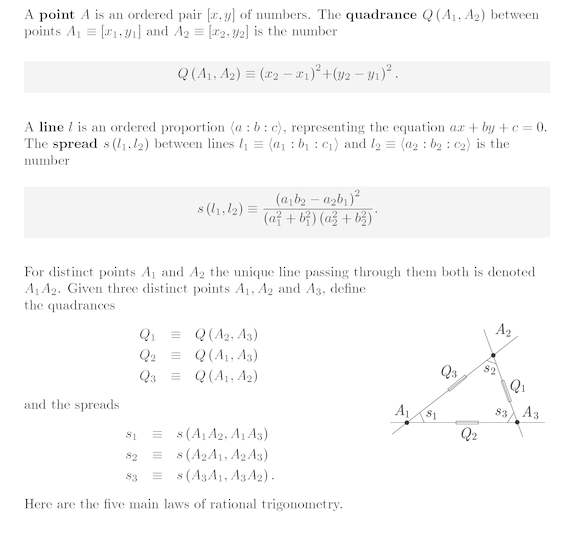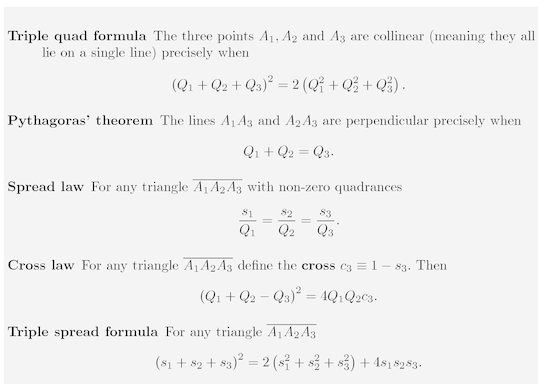• ms@ms.lt
• +370 607 27 665
• My work is in the Public Domain for all to share freely.

Introduction E9F5FC

Understandable FFFFFF

Questions FFFFC0

Notes EEEEEE

Software

Write down all of the results of Universal Hyperbolic Geometry. Organize them and interpret them in terms of

• matrices and symmetric functions of eigenvalues
• Lie theory (Lie bracket, Jacobi identity...)

Affine geometry

Lines are parallel:

• All (n 2) slopes the same.

Affine combinations

Vector is the relation between parallel lines.

Projective geometry

Space is divided into "infinity" and "finity".

Conformal geometry

Lines are perpendicular: Pythagorean theorem, inner product

Quadratic interpolations: law of cosines A2 - 2ABcos(theta) + B2 = C2 interpolation of (A-B)2 = C2, A2 + B2 = C2, (A+B)2 = C2. Consider triangles more generally. Or consider the function: A2 + B2 - C2 which is 0 when A and B are perpendicular, and nonzero 2ABcos(theta) otherwise.

Inner product defines the equation of a line and whether points lie on it. (a1, a2, a3)(x1, x2, 1) = 0.

Symplectic geometry

Oriented area, volume given by determinant.

• Quadrea: 4 x determinant squared. Determinant is positive three cycle and negative three cycle.
• Quadrea: Archimedes function (and when zero, we have collinearity by the Triple quad formula.
• Quadrea: Can repeatedly factor Archimedes function as a2-b2 = (a+b)(a-b) to get Heron's law for the area in terms of the lengths of the edges.What if we interpret the line as ax + by + cz = 0 where z=1 ?Why is the s1s2s3 term of the third power and not the second power?

#### UniversalHyperbolicGeometry

Naujausi pakeitimai

 Puslapis paskutinį kartą pakeistas 2018 vasario 04 d., 11:34